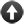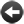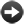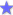§15.2. Numbers and real numbers

Inform uses two different kinds of numerical quantity: "number" and "real number". Neither is better than the other: they're different approaches, each good for a different purpose.

What Inform calls a "number" is a whole number, positive, negative or zero. The range of numbers we can hold is not unlimited - if the format Setting for a project is the Z-machine, then we have:

-32768, -32767, ..., -3, -2, -1, 0, 1, 2, 3, ..., 32767

and if it is set to Glulx, then we have:

-2147483648, -2147483647, ..., -3, -2, -1, 0, 1, 2, 3, ..., 2147483647

Numbers from zero to twelve may be written out, but larger ones must be written as numerals. So "twelve" or "12", but "13" only.

If we're using Glulx, Inform also has "real numbers" such as

2.1718, 4.0, -1633.9

which are not restricted to whole numbers, but which are stored only approximately: only about six to nine decimal digits can be relied on. For example,

showme 1.2345654321;
showme 1.2345667890;

produces

real number: 1.23457
real number: 1.23457

because these two numbers are so close together that Inform can't tell them apart. But we do also get the ability to represent enormously large or small quantities, and to help with that, Inform can read and write "scientific notation". For example,

let Avogadro's number be 6.022141 x 10^23;

is equivalent to typing

The "x 10^23" part tells Inform that the decimal point belongs 23 places to the left of where it's written. (In scientific papers, the 23 would be printed as a superscript -- it's 10 to the power 23 -- but that's not convenient to type in to the source text, so we use the "^" symbol to indicate superscript.) The range we can hold is roughly:

1.18 x 10^−38 to 3.4 x 10^38

It's hard to convey just how enormously different these two numbers are: if we used them to measure widths in meters, one would be a hundred trillion trillion times smaller than an atom, the other a billion times larger than the entire visible universe. Scientific notation is the ultimate adjustable spanner.

Inform also allows the two most famous real numbers in mathematics to be given by their names:

pi
e

which are close to 3.14159265 and 2.7182818 respectively. (Lower case letters must be used: these can't be written "Pi" or "E". Euler's constant gamma, always in the bronze medal position, will have to be written out longhand as 0.5772156649.)

Most computer programming languages traditionally write floating-point numbers using the E notation, like so:

6.022141E+23;

Inform will follow suit if the use option "Use engineering notation." is set, but by default it isn't.Start of Chapter 15: Numbers and EquationsBack to §15.1. How do we measure things?Onward to §15.3. Real number conversionsExampleAlias A telephone with phone numbers of the standard American seven-digit length.